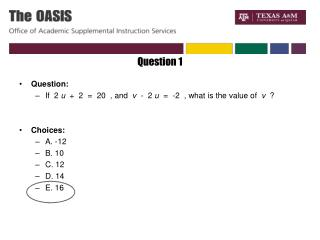DownloadDownload PresentationQuestion 1

# Question 1

Télécharger la présentation## Question 1

- - - - - - - - - - - - - - - - - - - - - - - - - - - E N D - - - - - - - - - - - - - - - - - - - - - - - - - - -
##### Presentation Transcript

1. Question 1 • Question: • If  2 u  +  2  =  20  , and  v  -  2 u  =  -2  , what is the value of  v  ?  • Choices: • A. -12 • B. 10 • C. 12 • D. 14 • E. 16

2. Question 2 • Question: • At a certain store, a 7.5 pound bag of rice costs \$4.80. What is the cost per ounce of rice? (There are 16 ounces in a pound.)  • Choices: • A. \$0.02 • B. \$0.03 • C. \$0.04 • D. \$0.05 • E. \$0.15

3. Question 3 • Question: • On Farm X, farm workers work 6 days per week and earn \$23.00 per day, plus \$0.74 per bushel filled. If a certain farm worker earned \$165.38 in a week, how many bushels did she fill in that week?  • Choices: • A. 7 • B. 31 • C. 37 • D. 68 • E. 192

4. Question 4 • Question: • A garden needs to be covered with grass sod. The garden has the dimensions shown above. How many square feet of sod are required to cover the garden?  • Choices: • A. 720 • B. 864 • C. 984 • D. 1,056 • E. 1,200

5. Question 5 • Question: • If x is a real number and 5x= 625 then 3 x 3x =? • Choices: • A. 5 • B. 9 • C. 45 • D. 125 • E. 243

6. Question 6 • Question: • A right triangle has sides of length 15, 20, and 25 meters. What is the area of the triangle in square meters?  • Choices: • A. 60 • B. 150 • C. 250 • D. 625 • E. 7,500

7. Question 7 • Question: • A store displays all the AA batteries it has in a display case on a wall. The batteries can be displayed in three columns of equal height. The batteries can also be displayed in four, five, or six columns of equal height. What is the smallest number of batteries that the store can have?   • Choices: • A. 15 • B. 30 • C. 60 • D. 180 • E. 360

8. Question 8 • Question: • Two sides of a triangle are 17 and 18 feet. Which of the following CANNOT be the length of the third side? • Choices: • A. 2 • B. 8 • C. 15 • D. 18 • E. 36